Courses

# Examples : Euler-Lagrange Equation (Part - 1) - Classical Mechanics, CSIR-NET Physical Sciences Physics Notes | EduRev

## Physics for IIT JAM, UGC - NET, CSIR NET

Created by: Akhilesh Thakur

## Physics : Examples : Euler-Lagrange Equation (Part - 1) - Classical Mechanics, CSIR-NET Physical Sciences Physics Notes | EduRev

The document Examples : Euler-Lagrange Equation (Part - 1) - Classical Mechanics, CSIR-NET Physical Sciences Physics Notes | EduRev is a part of the Physics Course Physics for IIT JAM, UGC - NET, CSIR NET.
All you need of Physics at this link: Physics

Example 1 : Show that the geodesic (shortest distance between two points) in a Euclidian plane is a straight line.

Solution: Take P ( x1 , y1 ) and Q ( x2 , y2 ) be two fixed points in a Euclidean plane. Let y = f ( x ) be the curve between P and Q. Then the element of distance between two neighboring points on the curve y = f ( x ) joining P and Q is given by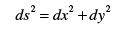Hence the total distance between the point P and Q along the curve is given by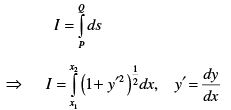. . . (1)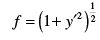. . . (2)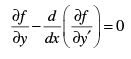. . . (3)

Now from equation (2) we find that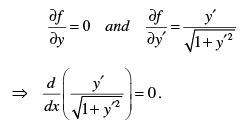Integrating we get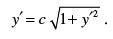Squaring we get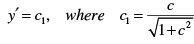Integrating we get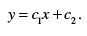. . . (4)

This is the required straight line. Thus the shortest distance between two points in a Euclidean plane is a straight line.

Example 2 : Show that the shortest distance between two polar points in a plane is a straight line.

Solution:  Define a curve in a plane. If A ( x, y ) and B ( x + dx, y + dy ) are infinitesimal points on the curve, then an element of distance between A and B is
given by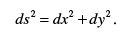. . . (1)

Let  θ = θ ( r ) be the polar equation of the curve and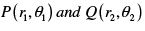be two polar points on it.  Recall the relations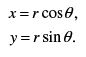Hence equation (1) becomes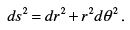. . . (2)

Thus the total distance between the points P and Q becomes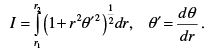. . . (3)

The functional I is shortest if the integrand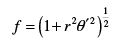. . . (4)

must satisfy the Euler-Lagrange’s differential equation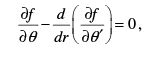...(5)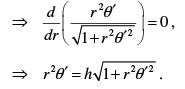Squaring and solving for θ we get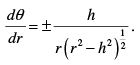On integrating we get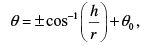where θ0 is a constant of integration. We write this as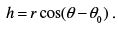. . . (6)

This is the polar form of the equation of straight line. Hence the shortest distance between two polar points is a straight line.

Note : If r = r (θ ) is the polar equation of the curve, then the length of the curve is given by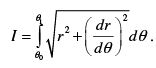Since the integrand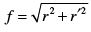does not contain θ , we therefore have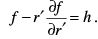Solving this equation we readily obtain the same polar equation of straight line as the geodesic.

Example 3 : Show that the geodesic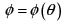on the surface of a sphere is an arc of the great circle.

Solution : Consider a sphere of radius r described by the equations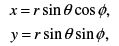. . . (1)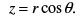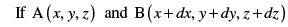be two neighboring points on the curve joining the points P and Q. Then the infinitesimal distance between A and B along the curve is given by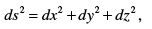. . . (2)

where from equation (1) we find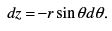. . . (3)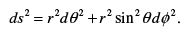. . . (4)

Hence the total distance between the points P and Q along the curve φ = φ (θ ) is given by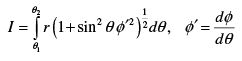where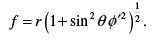. . . (6)

The curve is geodesic if the functional I is stationary. This is true if the function f must satisfy the Euler-Lagrange’s equations.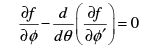. . . (7)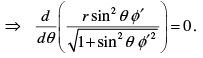Integrating we get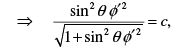Solving for φ ′ we get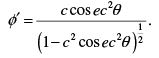On simplifying we get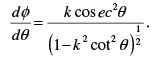. . . (8)

Put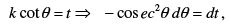Therefore we have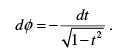Integrating we get

or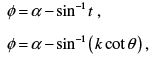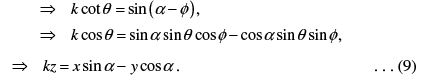This is the first-degree equation in x, y, z, which represents a plane. This plane passes through the origin, hence cutting the sphere in a great circle. Hence the geodesic on the surface of a sphere is an arc of a great circle.

Example 4 : Show that the curve is a catenary for which the area of surface of revolution is minimum when revolved about y-axis.

Solution: Consider a curve between two points ( x1 , y1 ) and ( x2 , y2 ) in the xy plane whose equation is y = y ( x ) . We form a surface by revolving the curve about y-axis. Our claim is to find the nature of the curve for which the surface area is minimum. Consider a small strip at a point A formed by revolving the arc length ds about y –axis. If the distance of the point A on the curve from y-axis is x, then the surface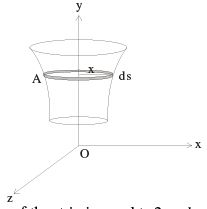area of the strip is equal to 2π x ds . But we know the element of arc ds is given by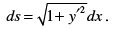Thus the surface area of the strip ds is equal to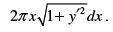Hence the total area of the surface of revolution of the curve y = y ( x ) about y- axis is given by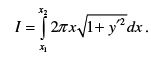. . . (1)

This surface area will be minimum if the integrand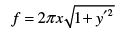. . . (2)

must satisfy Euler-Lagrange’s equation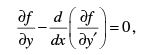. . . (3)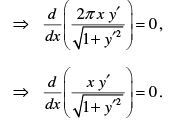Integrating we get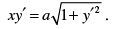Solving for y′ we get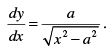Integrating we get

Or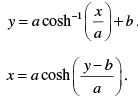. . . (4)

This shows that the curve is the catenary

• The Brachistochrone Problem :

The Brachistochrone is the curve joining two points not lie on the vertical line, such that the particle falling from rest under the influence of gravity from higher point to the lower point in minimum time. The curve is called the cycloid.

Example 5: Find the curve of quickest decent.

Or

A particle slides down a curve in the vertical plane under gravity. Find the curve such that it reaches the lowest point in shortest time.

Solution: Let A and B be two points on the curve not lie on the vertical line. Let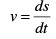be the speed of the particle along the curve. Then the time required to fall an
arc length ds is given by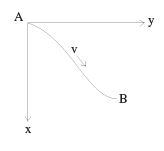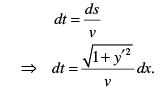Therefore the total time required for the particle to go from A to B is given by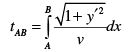. . . (1)

Since the particle falls freely under gravity, therefore its potential energy goes on decreasing and is given by

V = −mgx ,
and the kinetic energy is given by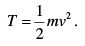Now from the principle of conservation of energy we have

T + V = constant.

Initially at point A, we have x = 0 and v = 0 . Hence the constant is zero.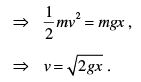. . . (2)

Hence equation (1) becomes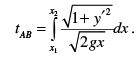. . . (3)

Thus tAB is minimum if the integrand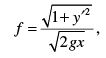. . . (4)

must satisfy Euler-Lagrange’s equation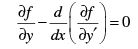. . . (5)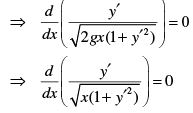Integrating we get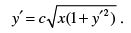Solving it for y′ we get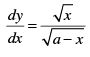Integrating we get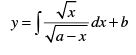. . . (6)

Put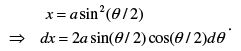. . . (7)

Hence

If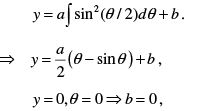hence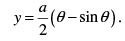. . . (8)

Thus from equations (7) and (8) we have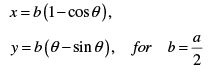This is a cycloid. Thus the curve is a cycloid for which the time of decent is minimum.

Example 6 : Find the extremal of the functional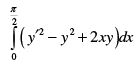subject to the conditions that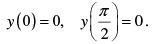Solution:  Let the functional be denoted by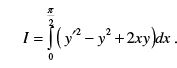. . . (1)

The functional is extremum if the integrand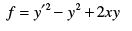. . . (2)

must satisfy the Euler-Lagrange’s equation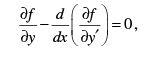. . . (3)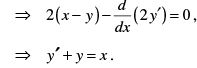. . . (4)

This is second order differential equation, whose complementary function (C.F.) is given by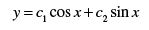. . . (5)

where c1 and c2 are arbitrary constants. The particular integral (P.I.) is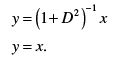Hence the general solution is given by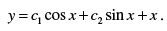. . . (6)

This shows that the extremals of the functional are the two-parameter family of curves. On using the boundary conditions we obtain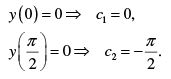Hence the required extremal is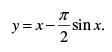. . . (7)

Example 7 : Find the extremal of the functional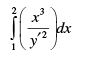subject to the conditions that

y (1) = 0, y ( 2 ) = 3 .

Solution:  Let the functional be denoted by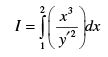. . . (1)

The functional is extremum if the integrand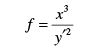. . . (2)

must satisfy the Euler-Lagrange’s equation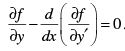(3)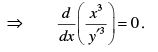Integrating we get

or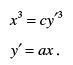Integrating we get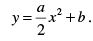. . . (4)

Now using the boundary conditions we get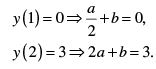Solving these two equations we obtain

a = 2, b = −1 .

Hence the required functional becomes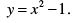. . . (5)

159 docs

,

,

,

,

,

,

,

,

,

,

,

,

,

,

,

,

,

,

,

,

,

,

,

,

;